Subsections

Features and their derivatives

Distance

Distance is defined by points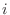and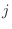: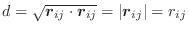(A.38)

where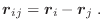(A.39)

The first derivatives of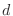with respect to Cartesian coordinates are: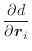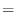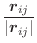(A.40)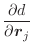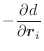(A.41)

Angle

Angle is defined by points,, and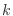, and spanned by vectors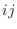and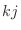: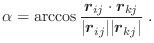(A.42)

It lies in the interval from 0 to 180°. Internal MODELLER units are radians.

The first derivatives of α with respect to Cartesian coordinates are: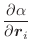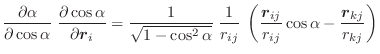(A.43)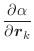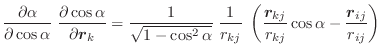(A.44)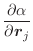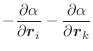(A.45)

These equations for the derivatives have a numerical instability when the angle goes to 0 or to 180°. Presently, the problem is ‘solved’ by testing for the size of the angle; if it is too small, the derivatives are set to 0 in the hope that other restraints will eventually pull the angle towards well behaved regions. Thus, angle restraints of 0 or 180° should not be used in the conjugate gradients or molecular dynamics optimizations.

Dihedral angle

Dihedral angle is defined by points,,, and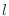(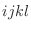):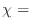sign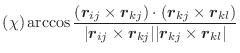(A.46)

where

 sign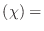sign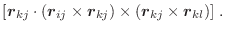(A.47)

The first derivatives of χ with respect to Cartesian coordinates are: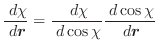(A.48)

where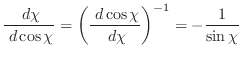(A.49)

and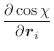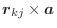(A.50)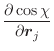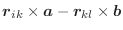(A.51)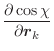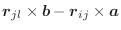(A.52)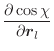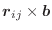(A.53)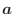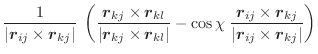(A.54)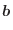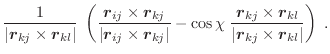(A.55)

These equations for the derivatives have a numerical instability when the angle goes to 0. Thus, the following set of equations is used instead [van Schaik et al., 1993]: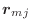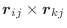(A.56)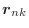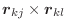(A.57)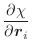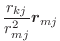(A.58)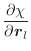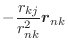(A.59)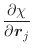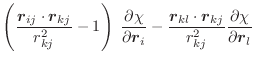(A.60)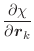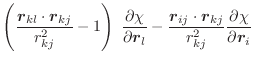(A.61)

The only possible instability in these equations is when the length of the central bond of the dihedral,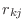, goes to 0. In such a case, which should not happen, the derivatives are set to 0. The expressions for an improper dihedral angle, as opposed to a dihedral or dihedral angle, are the same, except that indicesare permuted to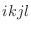. In both cases, covalent bonds,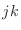, and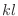are defining the angle.

Atomic solvent accessibility

This is the accessibility value calculated by the PSA algorithm (see model.write_data()). This is usually set by the last call to Restraints.make() or Restraints.make_distance(). First derivatives are not calculated, and are always returned as 0.

Atomic density

Atomic density for a given atom is simply calculated as the number of atoms within a distance energy_data.contact_shell of that atom. First derivatives are not calculated, and are always returned as 0.

Atomic coordinates

The absolute atomic coordinates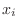,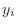and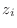are available for every point, primarily for use in anchoring points to planes, lines or points. Their first derivatives with respect to Cartesian coordinates are of course simply 0 or 1.

Automatic builds 2018-12-06